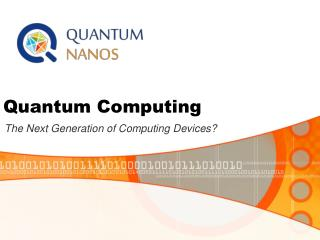DownloadDownload PresentationQuantum Computing

# Quantum Computing

Télécharger la présentation## Quantum Computing

- - - - - - - - - - - - - - - - - - - - - - - - - - - E N D - - - - - - - - - - - - - - - - - - - - - - - - - - -
##### Presentation Transcript

1. Quantum Computing The Next Generation of Computing Devices?

2. What is a Quantum Computer? • Quantum Computer • A computer that uses quantum mechanical phenomena to perform operations on data through devices such as superposition and entanglement. • Classical Computer (Binary) • A computer that uses voltages flowing through circuits and gates, which can be calculated entirely by classical mechanics.

3. The Need For Speed... • Classical Digital Computer • Moore’s Law: # of transistors on chip doubles every 18 months—microprocessor circuits will measure on atomic scale by 2020-2030 • Downscaling of circuit board layout/components is leading to discrepancies. • Copper traces are actually crystallizing and shorting out! • Emergence of quantum phenomena such as electrons tunneling through the barriers between wires. • Serial Processing – one operation at a time • 64-bit classical computer operates speeds measured in gigaflops (billions of floating-point operations per second). • Quantum Computer • Harnesses the power of atoms and molecules to perform memory and processing tasks • Parallel Processing – millions of operations at a time • 30-qubit quantum computer equals the processing powerof conventional computer that running at 10 teraflops(trillions of floating-point operations per second).

4. Classical vs Quantum Bits • Classical Bit • 2 Basic states – off or on: 0, 1 • Mutually exclusive • Quantum Bit (Qubit) • 2 Basic states – ket 0, ket 1: • Superposition of both states –(not continuous in nature) • Quantum entanglement • 2 or more objects must be described in reference to one another • Entanglement is a non-local property that allows a set of qubits to express superpositions of different binary strings (01010 and 11111, for example) simultaneously

5. Quantum Computing Power • Integer Factorization • Impossible for digital computers to factor large numbers which are the products of two primes of nearly equal size • Quantum Computer with 2n qubits can factor numbers with lengths of n bits (binary) • Quantum Database Search • Example: To search the entire Library of Congress for one’s name given an unsorted database... • Classical Computer – 100 years • Quantum Computer – ½ second

6. Practical Quantum Computer Applications • Quantum Mechanics Simulations • physics, chemistry, materials science, nanotechnology, biology and medicine. • Computer can compute millions of variables at once. • All are limited today by the slow speed of quantum mechanical simulations. • Cryptoanalysis • Capable of cracking extremely complicated codes • RSA encryption • Typically uses numbers with over 200 digits

7. Quantum Computing History • 1973 - Alexander Holevo publishes paper showing that n qubits cannot carry more than n classical bits of information. • 1976 - Polish mathematical physicist Roman Ingarden shows that Shannon information theory cannot directly be generalized to the quantum case. • 1981 - Richard Feynman determines that it is impossible to efficiently simulate a evolution of a quantum system on a classical computer. • 1985 - David Deutsch of the University of Oxford, describes the first universal quantum computer. • 1993 - Dan Simon, at Universite de Montreal, invents an oracle problem for which quantum computer would be exponentially faster than conventional computer. This algorithm introduced the main ideas which were then developed in Peter Shor's factoring algorithm. • 1994 - Peter Shor, at AT&T's Bell Labs discovers algorithm to allow quantum computers to factor large integers quickly. Shor's algorithm could theoretically break many of the cryptosystems in use today. • 1995 - Shorproposs the first scheme for quantum error correction. • 1996 - Lov Grover, at Bell Labs, invents quantum database search algorithm. • 1997 - David Cory, A.F. Fahmy, Timothy Havel, Neil Gershenfeld and Isaac Chuang publish the first papers on quantum computers based on bulk spin resonance, or thermal ensembles. Computers are actually a single, small molecule, storing qubits in the spin of protons and neutrons. Trillions of trillions of these can float in a cup of water. • 1998 - First working 2-qubit NMR computer demonstrated at University of California, Berkeley. • 1999 - First working 3-qubit NMR computer demonstrated at IBM's Almaden Research Center. First execution of Grover's algorithm. • 2000 - First working 5-qubit NMR computer demonstrated at IBM's Almaden Research Center. • 2001 - First working 7-qubit NMR computer demonstrated at IBM's Almaden Research Center.First execution of Shor's algorithm. The number 15 was factored using 1018 identicalmolecules, each containing 7 atoms.

8. Candidates for Quantum Computers • Superconductor-based quantum computers(including SQUID-based quantum computers) • Ion trap-based quantum computers • "Nuclear magnetic resonance on molecules in solution"-based • “Quantum dot on surface"-based • “Laser acting on floating ions (in vacuum)"-based (Ion trapping) • "Cavity quantum electrodynamics" (CQED)-based • Molecular magnet-based • Fullerene-based ESR quantum computer • Solid state NMR Kane quantum computer

9. Quantum Computing Problems • Current technology • ≈ 40 Qubit operating machine needed to rival current classical equivalents. • Errors • Decoherence - the tendency of a quantum computer to decay from a given quantum state into an incoherent state as it interacts with the environment. • Interactions are unavoidable and induce breakdown of information stored in the quantum computer resulting in computation errors. • Error rates are typically proportional to the ratio of operating time to decoherence time • operations must be completed much quicker than the decoherence time.

10. Research References • http://www.qubit.org • http://www.cs.caltech.edu/~westside/quantum-intro.html • http://computer.howstuffworks.com/quantum-computer1.htm • http://en.wikipedia.org/wiki/Quantum_computers • http://www.carolla.com/quantum/QuantumComputers.htm